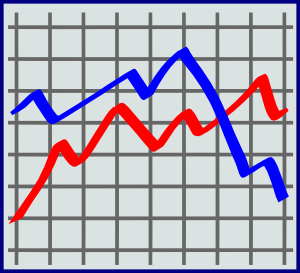## DATA MANAGEMENTUNIT: Data Management
BIG IDEAS (taken from “Big Ideas by Dr. Small”)
• To collect data, you must create appropriate questions and think about how best to gather the data.
• Graphs are powerful data displays since visual displays quickly reveal information about data.
• Bar graphs and pictographs are particularly useful for comparing the frequency of data in different categories. Line graphs are particularly useful for showing relationships between two quantities and trends.
• It is important for students not only to read information from graphs but to make inferences and draw conclusions.
• How the data is graphed (e.g. the use of different scales or intervals) can affect what conclusions are drawn from the data.
STUDENT LEARNING GOALS:
• I can collect unbiased primary and secondary, qualitative and quantitative, discrete and continuous data.
• I can draw and read pictographs, bar graphs, and line graphs
• I can choose the most appropriate graph for a set of data
• I can make conclusions and predictions from data in tables and graphs
CURRICULUM EXPECTATIONS:
• collect data by conducting a survey (e.g.,use an Internet survey tool) or an experiment to do with themselves, their environment, issues in their school or community, or content from another subject, and record observations or measurements;
• collect and organize discrete or continuous primary data and secondary data (e.g., electronic data from websites such as E-Stat or Census At Schools) and display the data in charts, tables, and graphs (including continuous line graphs) that have appropriate titles, labels (e.g., appropriate units marked on the axes), and scales (e.g., with appropriate increments) that suit the range and distribution of the data, using a variety of tools (e.g., graph paper, spreadsheets, dynamic statistical software);
• select an appropriate type of graph to represent a set of data, graph the data using technology, and justify the choice of graph (i.e., from types of graphs already studied, such as pictographs, horizontal or vertical bar graphs, stem-and-leaf plots, double bar graphs, broken-line graphs, and continuous line graphs);
• determine, through investigation, how well a set of data represents a population, on the basis of the method that was used to collect the data
• read, interpret, and draw conclusions from primary data (e.g., survey results, measure- ments, observations) and from secondary data (e.g., sports data in the newspaper, data from the Internet about movies), presented in charts, tables, and graphs (including continuous line graphs);
• compare, through investigation, different graphical representations of the same data
• explain how different scales used on graphs can influence conclusions drawn from the data
• demonstrate an understanding of mean (e.g., mean differs from median and mode because it is a value that “balances” a set of data – like the centre point or fulcrum in a lever), and use the mean to compare two sets of related data, with and without the use of technology
• demonstrate, through investigation, an understanding of how data from charts, tables, and graphs can be used to make inferences and convincing arguments (e.g., describe examples found in newspapers and magazines).
ONLINE PRACTICE QUIZZES:
ONLINE GAMES:

## PROBABILITYUNIT: Probability
BIG IDEAS (taken from “Big Ideas by Dr. Small”):
• Real-world situations can be represented using probabilities.
• Probability can be used to predict outcomes.
• An experimental probability is based on past events and experiments.
• A theoretical probability is based on an analysis of what could happen.
STUDENT LEARNING GOALS:
• I can identify probabilities from real-world scenarios.
• I can make predictions based on theoretical and/or experimental probabilities.
CURRICULUM EXPECTATIONS:
• express theoretical probability as a ratio of the number of favourable outcomes to the total number of possible outcomes, where all outcomes are equally likely (e.g., the theoretical probability of rolling an odd number on a six-sided number cube because, of six equally likely outcomes,
• represent the probability of an event (i.e.,the likelihood that the event will occur), using a value from the range of 0 (never happens or impossible) to 1 (always happens or certain);
• predict the frequency of an outcome of a simple probability experiment or game, by calculating and using the theoretical probability of that outcome
ONLINE PRACTICE QUIZZES (from Nelson Education):